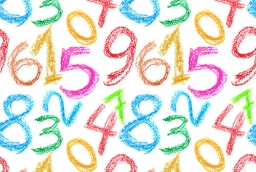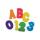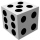# Divisible by nine

How many three-digit natural numbers in total are divisible without a remainder by the number 9?

n =  100

### Step-by-step explanation:Did you find an error or inaccuracy? Feel free to write us. Thank you!Tips to related online calculators
Do you want to perform natural numbers division - find the quotient and remainder?

## Related math problems and questions:

• NumberWhat number should be placed instead of the asterisk in number 702*8 to get a number divisible by 6?
• Twenty-fiveHow many are three-digit natural numbers divisible by 25?
• Divisibility by 12Replace the letters A and B by digits so that the resulting number x is divisible by twelve /find all options/. x = 2A3B How many are the overall solutions?
• SymmetryEva loves symmetry in shapes and numbers. Yesterday she invented a completely new kind of symmetry - divisible symmetry. She wrote all five-digit numbers with different digits with the following property: the first digit is divisible by 1, the second by 2
• By sixFrom the digits 1,2,3,4 we create the long integer number 123412341234. .. .. , which will have 962 digits. Is this number divisible by 6?
• 7 digit numberIf 3c54d10 is divisible by 330, what is the sum of c and d?
• Find unknown numberWhat is the number between 50 and 55 that is divisible by 2,3,6,9?
• Unknown integerFind the smallest integer: divided by 2, the remainder is 1. divided by 3, the remainder is 2. divided by 4, the remainder is 3. ... divided by eight, the remainder is 7, divided by 9 the remainder is 8.
• RemainderA is an arbitrary integer that gives remainder 1 in the division with 6. B is an arbitrary integer that gives remainder 2 the division by. What makes remainder in division by 3 product of numbers A x B ?
• Inverted nineIn the hotel Inverted Nine, each hotel room number is divisible by 6. How many rooms can we count with the three-digit number registered by digits 1,8,7,4,9?
• DivisibilityIs the number 761082 exactly divisible by 9? (the result is the integer and/or remainder is zero)
• Divisible by fourAre 240 divisible by 4?
• How manyHow many numbers are less than 222 with a digit sum is 8?
• NumbersDetermine the number of all positive integers less than 4183444 if each is divisible by 29, 7, 17. What is its sum?
• RemainderWhat is the remainder of the division of natural numbers 293 and 7?
• Result and remainderAfter dividing the unknown number by the number 23, the quotient 11 and the remainder 4 are formed. Find an unknown number.
• The diceFind the probability that a number divisible by two or three will fall when the dice are rolled.